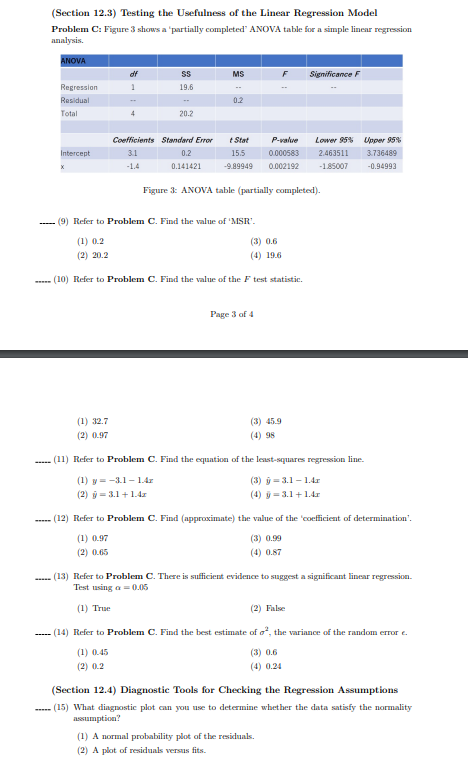Home / Expert Answers / Statistics and Probability / problem-c-section-12-3-testing-the-usefulness-of-the-linear-regression-model-problem-ca-figure-3-pa376

# (Solved): problem C (Section 12.3) Testing the Usefulness of the Linear Regression Model Problem Ca Figure 3 ...

problem C(Section 12.3) Testing the Usefulness of the Linear Regression Model Problem Ca Figure 3 shows a 'partially completed' ANOVA table for a simple linear regression analysis. Figure 3: ANOVA table (partially completed). (9) Refer to Problem C. Find the value of 'MSR'- (1) (3) (2) (4) (10) Refer to Problem C. Find the value of the test statistic. Page 3 of 4 (1) (3) (2) (4) 98 (11) Refer to Problem C. Find the equation of the lesst-squares regression line. (1) (3) (2) (4) .... (12) Refer to Problem C. Find (approximate) the value of the 'ooefficient of determination'. (1) (3) (2) (4) (13) Refer to Problem . There is sufficient evidence to suggest a significant linear regression. Test using (1) True (2) False ... (14) Refer to Problem C. Find the best estimate of , the variance of the random error . (1) (3) (2) (4) (Section 12.4) Diagnostic Tools for Checking the Regression Assumptions (15) What dingnostic plot can you use to determine whether the data satisfy the normality assumption? (1) A normal probability plot of the residuals. (2) A plot of residuals versus fits.

We have an Answer from Expert